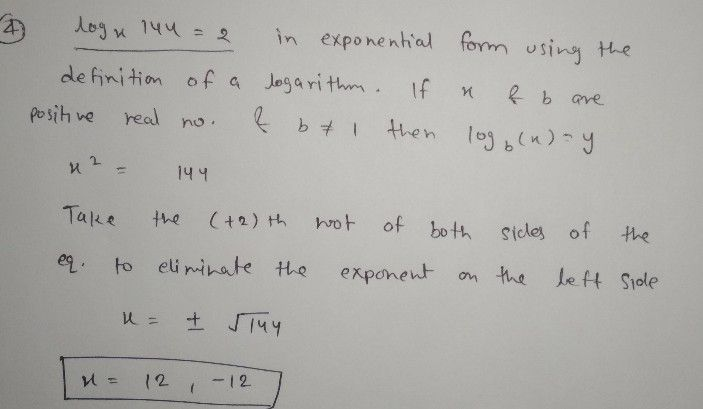Symbol
Problem$1$ $log _{3}\left(5x\right)=log _{3}50$ $2.$ $log _{4}\left(3x-1\right)=2$ $3,log _{3}\left(x+4\right)=log _{3}\left(2x-4\right)$ $4$ $log _{x}144=2$ $5$ $log _{4}\left(5x-1\right)>log _{4}\left(x+5\right)$ SULONG RduKALIOAD $1\right)$ SCHOOL ID 30aest PAe Steet ruy an Peoaro 6710 Caaye Prvi e Hench Unhe h Lect, Enower theog Decisbin
10th-13th grade
Other
Search count: 109
SolutionQanda teacher - AskSubhashhey dear feel free to ask queries
dear here only one question allowed
it's against quandas policy
I hope you understand
Please give 3000 coins as thank you gift this will not reduce your coins
happy learning ☺️Student
oh thank u for answering my questions
what about 1 23 5 queston sirQanda teacher - AskSubhash
dear here only one question allowed
it's against quandas policy
plz understand